# http www comp nus edu sgcs 2100 Lecture

• Slides: 25http: //www. comp. nus. edu. sg/~cs 2100/ Lecture #13 Boolean Algebra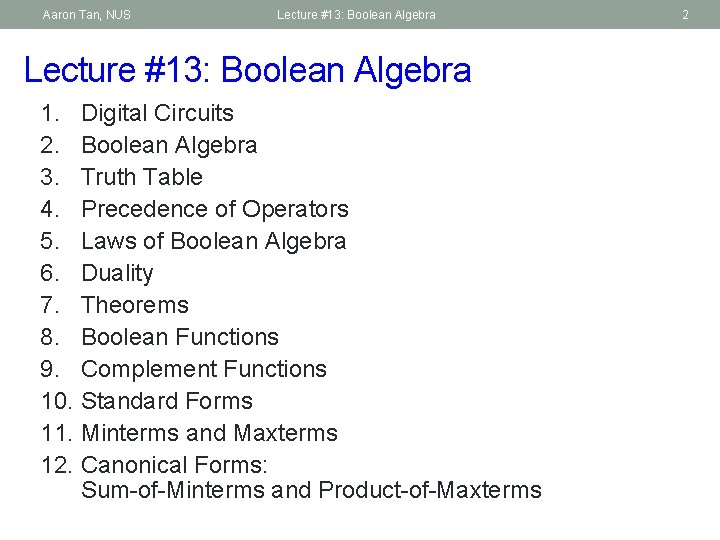Aaron Tan, NUS Lecture #13: Boolean Algebra 1. Digital Circuits 2. Boolean Algebra 3. Truth Table 4. Precedence of Operators 5. Laws of Boolean Algebra 6. Duality 7. Theorems 8. Boolean Functions 9. Complement Functions 10. Standard Forms 11. Minterms and Maxterms 12. Canonical Forms: Sum-of-Minterms and Product-of-Maxterms 2Aaron Tan, NUS Lecture #13: Boolean Algebra 3 1. Digital Circuits (1/2) § Two voltage levels § High/true/1/asserted § Low/false/0/deasserted High Low Signals in digital circuit Signals in analog circuit § Advantages of digital circuits over analog circuits § More reliable (simpler circuits, less noise-prone ) § Specified accuracy (determinable) § Abstraction can be applied using simple mathematical model – Boolean Algebra § Ease design, analysis and simplification of digital circuit – Digital Logic Design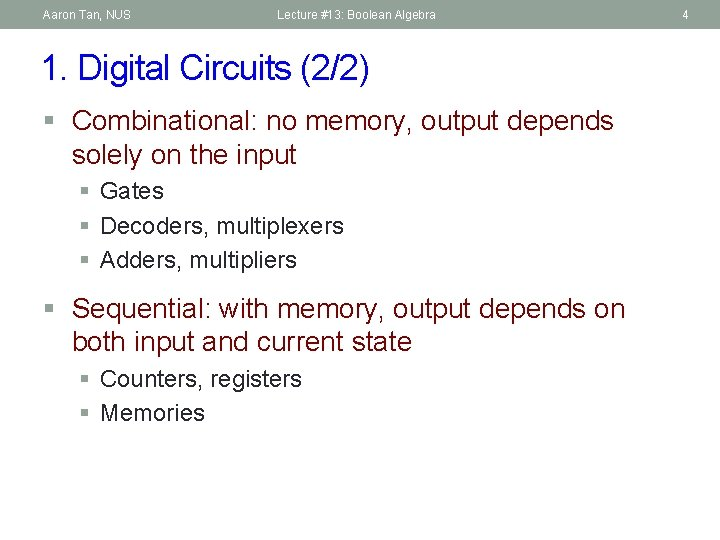Aaron Tan, NUS Lecture #13: Boolean Algebra 1. Digital Circuits (2/2) § Combinational: no memory, output depends solely on the input § Gates § Decoders, multiplexers § Adders, multipliers § Sequential: with memory, output depends on both input and current state § Counters, registers § Memories 4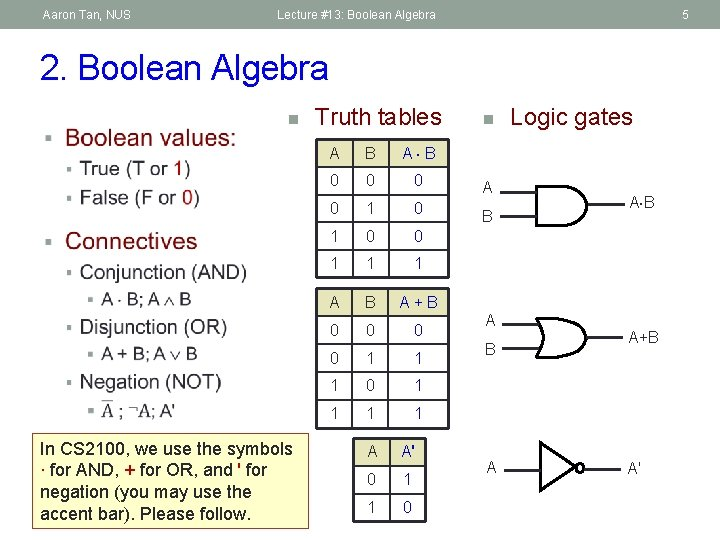Aaron Tan, NUS Lecture #13: Boolean Algebra 5 2. Boolean Algebra n In CS 2100, we use the symbols ∙ for AND, + for OR, and ' for negation (you may use the accent bar). Please follow. Truth tables A B A B 0 0 1 1 1 A B A + B 0 0 1 1 1 0 1 1 A A' 0 1 1 0 n A B A Logic gates A B A+B A'Aaron Tan, NUS Lecture #13: Boolean Algebra 6 2. Boolean Algebra: AND § Do write the AND operator ∙ (instead of omitting it) § Example: Write a∙b instead of ab § Why? Writing ab could mean that it is a 2 -bit value.Aaron Tan, NUS Lecture #13: Boolean Algebra 7 3. Truth Table § Provide a listing of every possible combination of inputs and its corresponding outputs. § Inputs are usually listed in binary sequence. § Example § Truth table with 3 inputs x, y, z and 2 outputs (y + z) and (x (y + z)) x y z y+z x (y + z) 0 0 0 0 1 1 0 0 1 1 1 0 0 0 0 1 1 1 1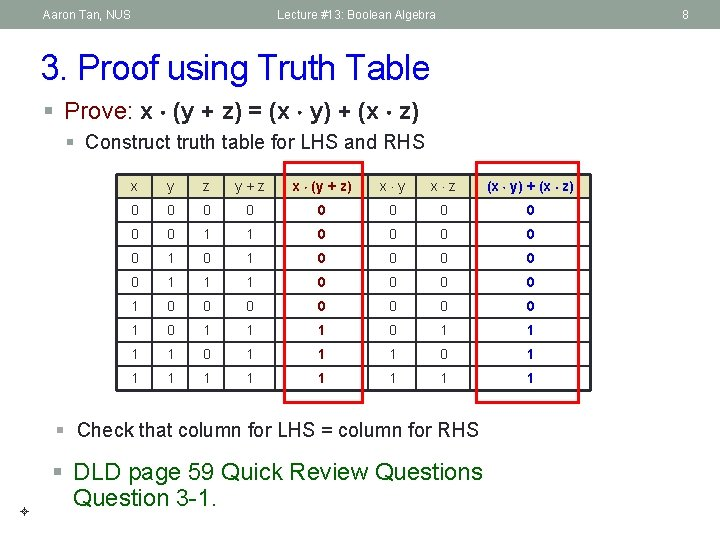Aaron Tan, NUS Lecture #13: Boolean Algebra 8 3. Proof using Truth Table § Prove: x (y + z) = (x y) + (x z) § Construct truth table for LHS and RHS x y z y + z x (y + z) x y x z (x y) + (x z) 0 0 0 0 0 1 1 0 0 0 1 1 1 0 0 0 0 1 1 1 0 1 1 1 1 1 § Check that column for LHS = column for RHS § DLD page 59 Quick Review Questions Question 3 -1.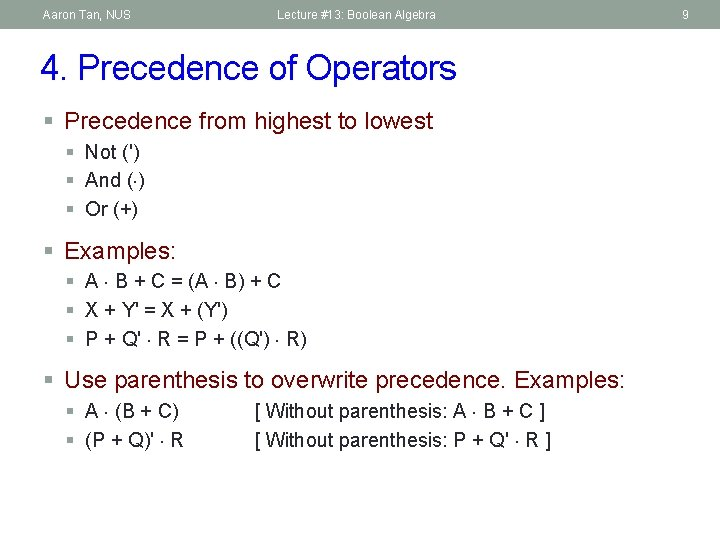Aaron Tan, NUS Lecture #13: Boolean Algebra 4. Precedence of Operators § Precedence from highest to lowest § Not (') § And ( ) § Or (+) § Examples: § A B + C = (A B) + C § X + Y' = X + (Y') § P + Q' R = P + ((Q') R) § Use parenthesis to overwrite precedence. Examples: § A (B + C) § (P + Q)' R [ Without parenthesis: A B + C ] [ Without parenthesis: P + Q' R ] 9Aaron Tan, NUS Lecture #13: Boolean Algebra 5. Laws of Boolean Algebra Identity laws A + 0 = 0 + A = A A 1 = 1 A = A Inverse/complement laws A + A' = 1 A A' = 0 Commutative laws A + B = B + A A B = B A Associative laws A + (B + C) = (A + B) + C A (B C) = (A B) C Distributive laws A (B + C) = (A B) + (A C) A + (B C) = (A + B) (A + C) 10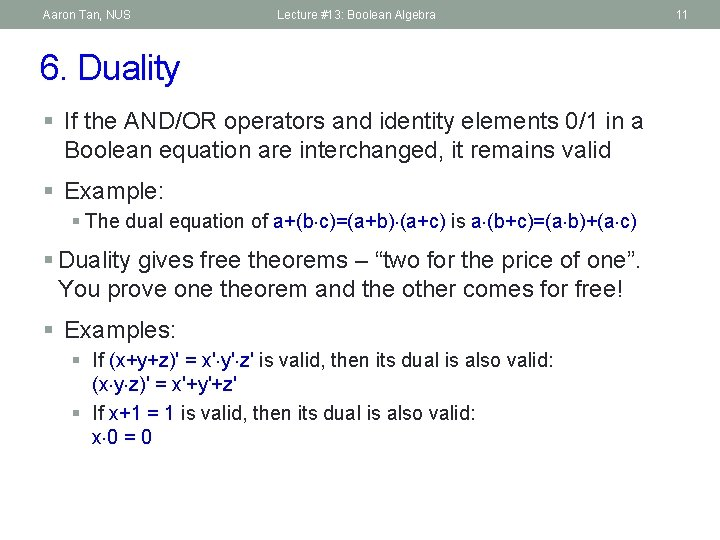Aaron Tan, NUS Lecture #13: Boolean Algebra 6. Duality § If the AND/OR operators and identity elements 0/1 in a Boolean equation are interchanged, it remains valid § Example: § The dual equation of a+(b c)=(a+b) (a+c) is a (b+c)=(a b)+(a c) § Duality gives free theorems – “two for the price of one”. You prove one theorem and the other comes for free! § Examples: § If (x+y+z)' = x' y' z' is valid, then its dual is also valid: (x y z)' = x'+y'+z' § If x+1 = 1 is valid, then its dual is also valid: x 0 = 0 11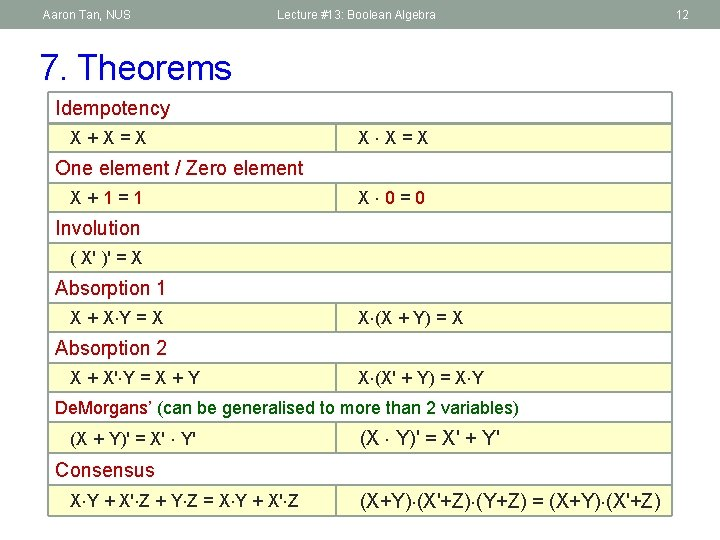Aaron Tan, NUS Lecture #13: Boolean Algebra 7. Theorems Idempotency X + X = X X X = X One element / Zero element X + 1 = 1 X 0 = 0 Involution ( X' )' = X Absorption 1 X + X Y = X X (X + Y) = X Absorption 2 X + X' Y = X + Y X (X' + Y) = X Y De. Morgans’ (can be generalised to more than 2 variables) (X + Y)' = X' Y' (X Y)' = X' + Y' Consensus X Y + X' Z + Y Z = X Y + X' Z (X+Y) (X'+Z) (Y+Z) = (X+Y) (X'+Z) 12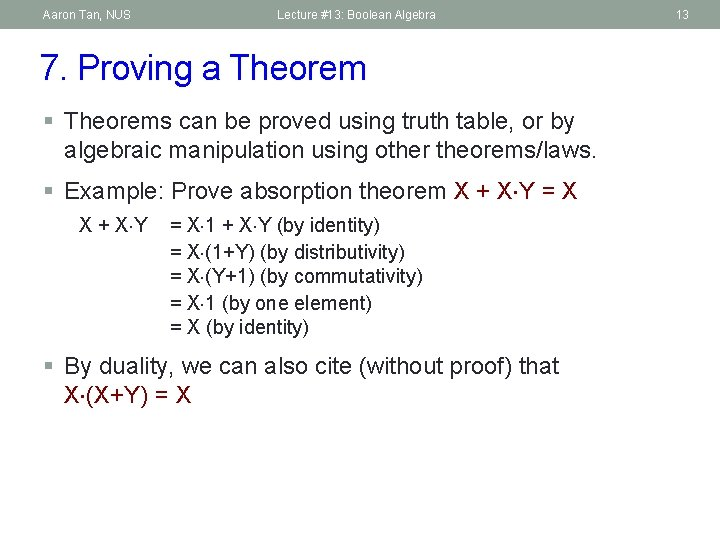Aaron Tan, NUS Lecture #13: Boolean Algebra 7. Proving a Theorem § Theorems can be proved using truth table, or by algebraic manipulation using other theorems/laws. § Example: Prove absorption theorem X + X Y = X X + X Y = X 1 + X Y (by identity) = X (1+Y) (by distributivity) = X (Y+1) (by commutativity) = X 1 (by one element) = X (by identity) § By duality, we can also cite (without proof) that X (X+Y) = X 13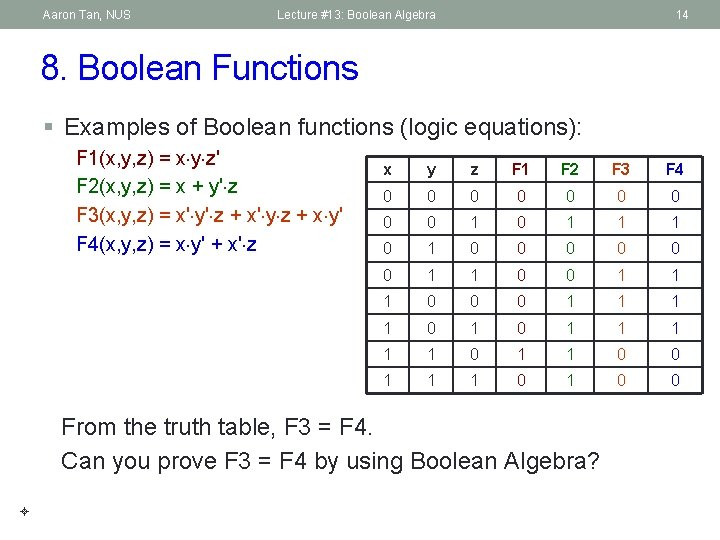Aaron Tan, NUS Lecture #13: Boolean Algebra 14 8. Boolean Functions § Examples of Boolean functions (logic equations): F 1(x, y, z) = x y z' F 2(x, y, z) = x + y' z F 3(x, y, z) = x' y' z + x' y z + x y' F 4(x, y, z) = x y' + x' z x y z F 1 F 2 F 3 F 4 0 0 0 0 0 1 1 1 0 0 0 0 1 1 1 0 0 0 1 1 1 0 1 1 0 0 1 1 1 0 0 From the truth table, F 3 = F 4. Can you prove F 3 = F 4 by using Boolean Algebra?Aaron Tan, NUS Lecture #13: Boolean Algebra 15 9. Complement Functions § Given a Boolean function F, the complement of F, denoted as F', is obtained by interchanging 1 with 0 in the function’s output values. § Example: F 1 = x y z' x y z F 1' § What is F 1' ? 0 0 1 0 1 0 1 1 0 0 0 1 1 0 1 0 1 1 1 0 1 § F 1' = (x y z')' = x' + y' + (z')' (De. Morgan’s) = x' + y' + z (Involution)Aaron Tan, NUS Lecture #13: Boolean Algebra 10. Standard Forms (1/2) § Certain types of Boolean expressions lead to circuits that are desirable from an implementation viewpoint. § Two standard forms: § Sum-of-Products (SOP) § Product-of-Sums (POS) § Literals § A Boolean variable on its own or in its complemented form § Examples: (1) x, (2) x', (3) y, (4) y' § Product term § A single literal or a logical product (AND) of several literals § Examples: (1) x, (2) x y z', (3) A' B, (4) A B, (5) d g' v w 16Aaron Tan, NUS Lecture #13: Boolean Algebra 10. Standard Forms (2/2) § Sum term § A single literal or a logical sum (OR) of several literals § Examples: (1) x, (2) x+y+z', (3) A'+B, (4) A+B, (5) c+d+h'+j § Sum-of-Products (SOP) expression § A product term or a logical sum (OR) of several product terms § Examples: (1) x, (2) x + y z', (3) x y' + x' y z, (4) A B + A' B', (5) A + B' C + A C' + C D § Product-of-Sums (POS) expression § A sum term or a logical product (AND) of several sum terms § Examples: (1) x, (2) x (y+z'), (3) (x+y') (x'+y+z), (4) (A+B) (A'+B'), (5) (A+B+C) D' (B'+D+E') § Every Boolean expression can be expressed in SOP or POS form. § DLD page 59 Quick Review Questions 3 -2 to 3 -5. 17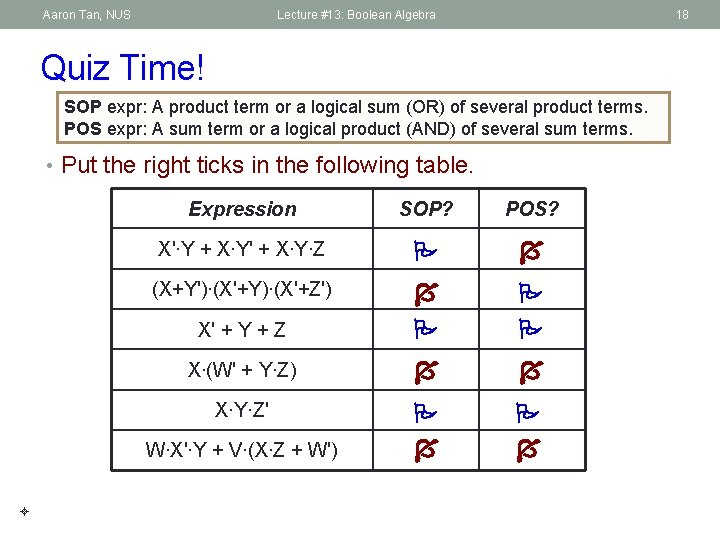Aaron Tan, NUS Lecture #13: Boolean Algebra 18 Quiz Time! SOP expr: A product term or a logical sum (OR) of several product terms. POS expr: A sum term or a logical product (AND) of several sum terms. • Put the right ticks in the following table. Expression SOP? POS? X'∙Y + X∙Y' + X∙Y∙Z (X+Y')∙(X'+Y)∙(X'+Z') X' + Y + Z X∙(W' + Y∙Z) X∙Y∙Z' W∙X'∙Y + V∙(X∙Z + W')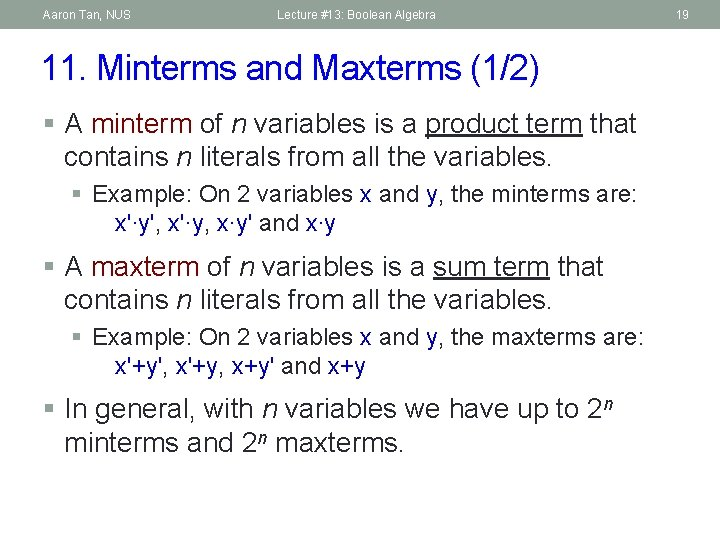Aaron Tan, NUS Lecture #13: Boolean Algebra 11. Minterms and Maxterms (1/2) § A minterm of n variables is a product term that contains n literals from all the variables. § Example: On 2 variables x and y, the minterms are: x'∙y', x'∙y, x∙y' and x∙y § A maxterm of n variables is a sum term that contains n literals from all the variables. § Example: On 2 variables x and y, the maxterms are: x'+y', x'+y, x+y' and x+y § In general, with n variables we have up to 2 n minterms and 2 n maxterms. 19Aaron Tan, NUS Lecture #13: Boolean Algebra 20 11. Minterms and Maxterms (2/2) § The minterms and maxterms on 2 variables are denoted by m 0 to m 3 and M 0 to M 3 respectively. x y 0 Minterms Maxterms Term Notation 0 x'∙y' m 0 x+y M 0 0 1 x'∙y m 1 x+y' M 1 1 0 x∙y' m 2 x'+y M 2 1 1 x∙y m 3 x'+y' M 3 § Each minterm is the complement of the corresponding maxterm § Example: m 2 = x∙y' m 2' = ( x∙y' )' = x' + ( y' )' = x' + y = M 2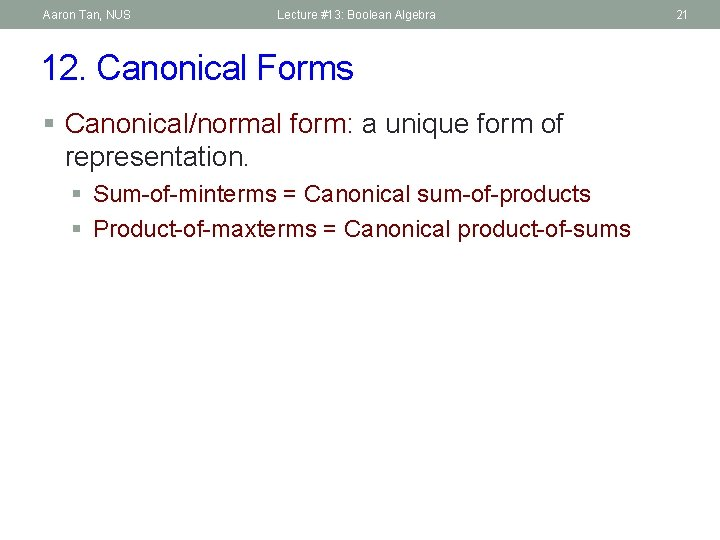Aaron Tan, NUS Lecture #13: Boolean Algebra 12. Canonical Forms § Canonical/normal form: a unique form of representation. § Sum-of-minterms = Canonical sum-of-products § Product-of-maxterms = Canonical product-of-sums 21Aaron Tan, NUS Lecture #13: Boolean Algebra 22 12. 1 Sum-of-Minterms § Given a truth table, example: § Obtain sum-of-minterms expression by gathering the minterms of the function (where output is 1). F 1 = x∙y∙z' = m 6 x y z F 1 F 2 F 3 0 0 0 0 1 0 1 0 0 0 1 1 1 0 1 1 1 0 F 2 = x'∙y'∙z + x∙y'∙z' + x∙y'∙z + x∙y∙z' + x∙y∙z = m 1 + m 4 + m 5 + m 6 + m 7 = Sm(1, 4, 5, 6, 7) or Sm(1, 4 – 7) F 3 = x'∙y'∙z + x'∙y∙z + x∙y'∙z' + x∙y'∙z = m 1 + m 3 + m 4 + m 5 = Sm(1, 3, 4, 5) or Sm(1, 3 – 5)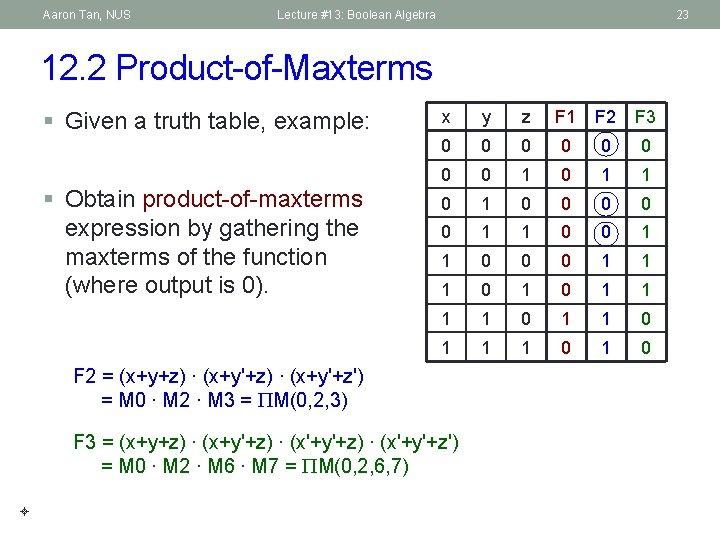Aaron Tan, NUS Lecture #13: Boolean Algebra 23 12. 2 Product-of-Maxterms § Given a truth table, example: § Obtain product-of-maxterms expression by gathering the maxterms of the function (where output is 0). x y z F 1 F 2 F 3 0 0 0 0 1 0 1 0 0 0 1 1 1 0 1 1 1 0 F 2 = (x+y+z) ∙ (x+y'+z') = M 0 ∙ M 2 ∙ M 3 = PM(0, 2, 3) F 3 = (x+y+z) ∙ (x+y'+z) ∙ (x'+y'+z') = M 0 ∙ M 2 ∙ M 6 ∙ M 7 = PM(0, 2, 6, 7)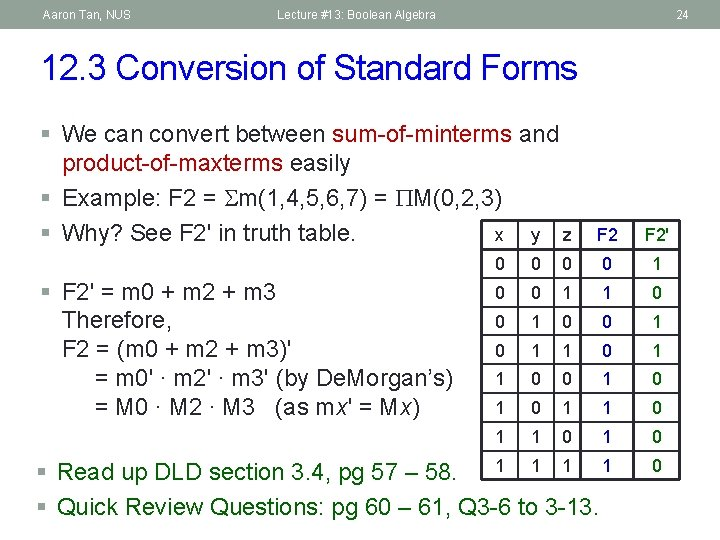Aaron Tan, NUS Lecture #13: Boolean Algebra 24 12. 3 Conversion of Standard Forms § We can convert between sum-of-minterms and product-of-maxterms easily § Example: F 2 = Sm(1, 4, 5, 6, 7) = PM(0, 2, 3) § Why? See F 2' in truth table. x y z F 2' 0 0 1 0 0 1 1 0 0 1 0 1 1 1 1 § Read up DLD section 3. 4, pg 57 – 58. § Quick Review Questions: pg 60 – 61, Q 3 -6 to 3 -13. 0 § F 2' = m 0 + m 2 + m 3 Therefore, F 2 = (m 0 + m 2 + m 3)' = m 0' ∙ m 2' ∙ m 3' (by De. Morgan’s) = M 0 ∙ M 2 ∙ M 3 (as mx' = Mx)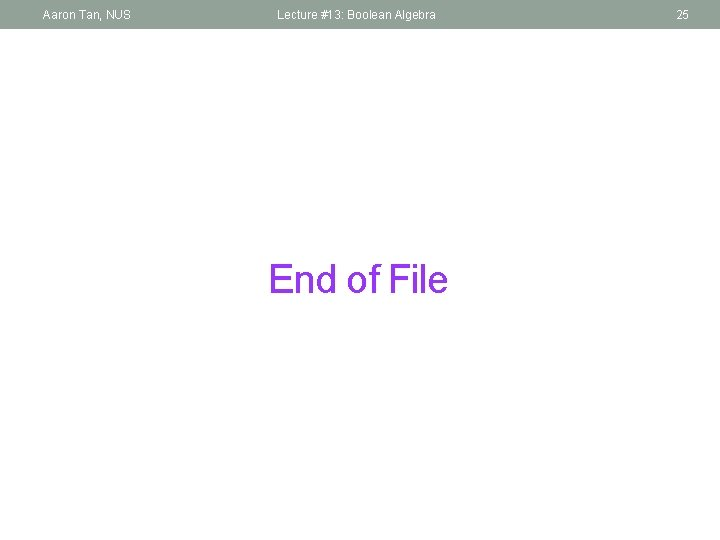Aaron Tan, NUS Lecture #13: Boolean Algebra End of File 25